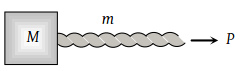A block of mass M is pulled along a horizontal frictionless surface by a rope of mass m. If a force P is applied at the free end of the rope, the force exerted by the rope on the block will be

(1) P

(2) $\frac{Pm}{M+m}$

(3) $\frac{PM}{M+m}$

(4) $\frac{Pm}{M-m}$

Concept Videos :-

#7-Solved-Examples-1
#8-Solved-Examples-2
#9-Solved-Examples-3
#10-Solved-Examples-4
#11-Solved-Examples-5
#12-Solved-Examples-6

Concept Questions :-

Application of laws

(3)Acceleration of the system $=\frac{P}{m+M}$

The force exerted by rope on the mass $=\frac{MP}{m+M}$

Difficulty Level:

• 15%
• 32%
• 52%
• 3%